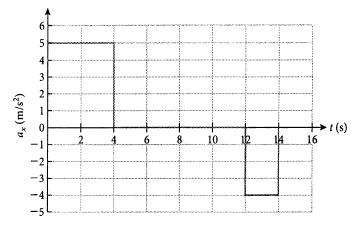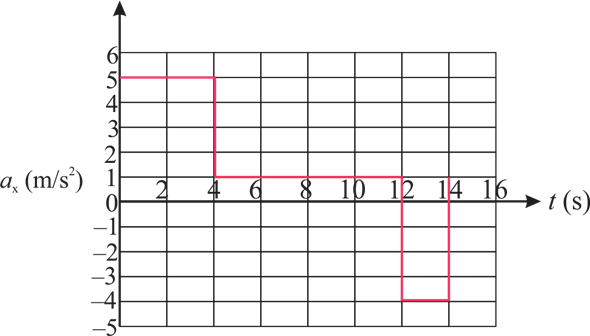Problem

# A motorcycle starts from rest and accelerates  as shown in the figure. Determine (a) the m...

A motorcycle starts from rest and accelerates  as shown in the figure. Determine (a) the motorcycle’s speed at t = 4.00 s and at t = 14.0 s, and (b)  the distance  traveled in the first 14.0 s.#### Step-by-Step Solution

Solution 1

This problem can be solved by making use of the values shown in the graph below.

The graph between acceleration and time:(a)

The speed of the motor cycle at $$t=4.00 \mathrm{~s}$$ is calculated by using the values in the above graph. The initial velocity of the motor cycle, $$v_{0}$$ is $$0 \mathrm{~m} / \mathrm{s}$$, time interval $$t$$ is $$4.00 \mathrm{~s}$$ and acceleration of the motorcycle $$a$$ is $$5 \mathrm{~m} / \mathrm{s}^{2}$$.

The speed of the motorcycle is,

\begin{aligned} v_{\mathrm{att}=4.0 \mathrm{~s}} &=v_{0}+a t \\ &=0+\left(5 \mathrm{~m} / \mathrm{s}^{2}\right)(4.0 \mathrm{~s}) \\ &=20 \mathrm{~m} / \mathrm{s} \end{aligned}

Thus, the speed of the motorcycle is $$20 \mathrm{~m} / \mathrm{s}$$.

The speed is constant between $$t=4.0 \mathrm{~s}$$ and $$t=12.0 \mathrm{~s} . \mathrm{So}$$, at $$t=12.00 \mathrm{~s}$$, the speed of the motorcycle is $$v=20 \mathrm{~m} / \mathrm{s}$$

To determine the speed at $$t=14.00 \mathrm{~s}$$, let us consider the time interval from $$t=12.0 \mathrm{~s}$$ to $$t=14.0 \mathrm{~s}$$, and the acceleration of the motorcycle is this time interval is, $$a=-4 \mathrm{~m} / \mathrm{s}^{2}$$.

The speed of the motorcycle is,

\begin{aligned} v_{\text {at t= } 14.0 s} &=v_{0}+a t \\ &=20 \mathrm{~m} / \mathrm{s}+\left(-4 \mathrm{~m} / \mathrm{s}^{2}\right)(14.0 \mathrm{~s}-12.0 \mathrm{~s}) \\ &=12 \mathrm{~m} / \mathrm{s} \end{aligned}

Thus, the speed of the motorcycle is $$12 \mathrm{~m} / \mathrm{s}$$.

(b)

To determine the distance traveled in the first $$14.0 \mathrm{~s}$$, Calculate the distance in the subsequent time intervals and then sum all the terms and the total distance is.

Distance traveled by the motorcycle from $$t=0 \mathrm{~s}$$ to $$t=4.0 \mathrm{~s}$$.

\begin{aligned} s_{1} &=v_{0} t+\frac{1}{2} a t^{2} \\ &=0+\frac{1}{2}\left(5 \mathrm{~m} / \mathrm{s}^{2}\right)(4.0 \mathrm{~s})^{2} \\ &=40 \mathrm{~m} \end{aligned}

Distance traveled by the motorcycle from $$t=4 \mathrm{~s}$$ to $$t=12.0 \mathrm{~s}$$,

\begin{aligned} s_{2} &=v t \\ &=(20 \mathrm{~m} / \mathrm{s})(12.0 \mathrm{~s}-4.0 \mathrm{~s}) \\ &=160 \mathrm{~m} \end{aligned}

Distance traveled by the motorcycle from $$t=12 \mathrm{~s}$$ to $$t=14.0 \mathrm{~s}$$,

\begin{aligned} s_{3} &=v_{0} t+\frac{1}{2} a t^{2} \\ &=(20 \mathrm{~m} / \mathrm{s})(14.0 \mathrm{~s}-12.0 \mathrm{~s})+\frac{1}{2}\left(-4 \mathrm{~m} / \mathrm{s}^{2}\right)(14.0 \mathrm{~s}-12.0 \mathrm{~s})^{2} \\ &=32 \mathrm{~m} \end{aligned}

Distance traveled in the first $$14.0 \mathrm{~s}$$ is

\begin{aligned} s &=s_{1}+s_{2}+s_{3} \\ &=40 \mathrm{~m}+160 \mathrm{~m}+32 \mathrm{~m} \\ &=232 \mathrm{~m} \end{aligned}